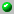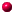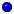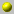Andrei Okounkov

The homepage can be accessed here.
• Office: 411 Eckhart Hall
• Address: Department of Mathematics, The University of Chicago, 5734 University Avenue, Chicago, IL 60637-1546
• Phone: (773) 702-7394, Fax: (773) 702-9787
• Email: okounkov@math.uchicago.edu

the following papers are available on our server= dvi format= ps format= tex format= pdf formatasymptotics_of_jack_polynomials_as_the_number_of_variables_goes_to_infinitybinomial_formula_for_macdonald_polynomials_and_its_applicationscb_n_type_interpolation_macdonald_polynomials_and_binomial_formula_for_koornwinder_polynomialson_newton_interpolation_of_symmetric_functionon_newton_interpolation_of_symmetric_functions_a_characterization_of_interpolation_macdonald_polynomialson_the_representations_of_the_infinite_symmetric_groupquantum_immanants_and_higher_capelli_identitiesshifted_jack_polynomials_binomial_formula_and_applications(shifted)_macdonald_polynomials_q_integral_representation_and_combinatorial_formulashifted_macdonald_polynomials_with_three_parameters_and_bionomial_formula_for_koornwinder_polynomialsshifted_macdonald_polynomials_with_three_parameters_and_bionomial_formula_for_koornwinder_polynomialsshifted_schur_functions_2_binomial_formula_for_characters_of_classical_groups_and_applicationsshifted_schur_functionsthe_boundary_of_young_graph_with_jack_edge_multiplicitiesthe_character_of_the_infinite_wedge_representationyoung_basis_wick_formula_and_higher_capelli_identities

Go back to the directory of pre and reprints.
Or search the database by keywords
Or look for the newest entires in the database